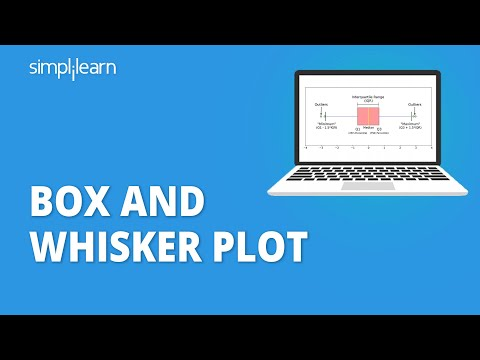### Tutorial PlaylistStatistics is the branch of science that deals with data collection, interpretation, and validation. The procedure of performing various statistical operations is known as statistical data analysis. One such method will be covered in this tutorial.

## What Are Box and Whisker Plot?

They are graphical representations of variation in a set of data. A histogram analysis is usually sufficient, but a box and plot can add more detail while also allowing multiple sets of data to be displayed in the same graph.• Median (Q2/ 50th percentile): The middle value of the dataset
• First Quartile (Q1): The 25th percentile of the dataset
• Third Quartile (Q3): The 75th percentile of the dataset

#### Post Graduate Program In Data Science

The Ultimate Ticket To Top Data Science Job Roles## Why Use a Box and Whisker Plot?

The box and plot is used when:

• You want to summarize the data from multiple sources and display the result in a single graph.
• You can compare the data from different categories using box and whiskers plots, making the decision-making easier and more effective.

## When to Use Box and Whisker Plot?

When you have multiple data sets from different sources that are related in some way, use boxes and plots. Here are some examples:

• Test results from different schools or classrooms
• Data from two identical machines producing the same goods

To make an accurate box and whiskers plot, you have to understand the five-number summary. The five-number summary is useful to identify and plot the points.

### Five Number Summary

The method to develop a box and plot comes from the five statistics below.

Minimum value: It is the smallest value of the data set.

Second quartile: The value below which the lower 25% of the data are contained.

Median value: The middle number in a range of numbers.

Third quartile: The value above which the upper 25% of the data are contained.

Maximum value: It is the largest value in the data set.

Now that you know about the five-number summary and the important points, let’s look at the steps to create a box and whiskers plot.

## How to Create Box and Whisker Plot?

Now, you will understand the steps involved in making a box and plot.

Consider the following example:

The sample age of 10 students at a training center are given below:

15, 27, 19, 18, 24, 19, 20, 25, 25, 28

Step1: Order the data from smallest to largest.

15, 18, 19, 19, 20, 24, 25, 25, 27, 28

Step 2: Find the median.

Since you have an even number of age values, the median is the mean of (n/2) and (n/2 + 1)th observation.

15, 18, 19, 19, 20, 24, 25, 25, 27, 28Step 3: Find the quartiles.

The first quartile is the median of the age values to the left of the median.

15, 18, 19, 19, 20

Q1 = 19

The third quartile is the median of the age values to the right of the median.

24, 25, 25, 27, 28

Q3 = 25

#### Free Course: Introduction to Data Science

Learn the Fundamentals of Data ScienceStep 4: Complete the five-number summary by finding the min and max values.

The min is the smallest age value, which is 15.

The max is the largest age value, which is 28.

The five-number summary is 15, 19, 22, 25, 28

## Making a Box Plot

Step 1:  Scale and label an axis that fits the five-number summary.Step 2: Draw whiskers from Q1 to the minimum age value and from Q3 to the maximum age value.The resulting graph gives you the idea of the minimum, lower quartile, median, upper quartile, and maximum age value of the students in the dataset.

Below are the key points that you can draw from the above box and plot:

• The lowest age among the students at the training center is 15 excluding outliers.
• About 25% of students are under 19 years of age.
• Close to 50% of the students are between 19 and 25 years of age.
• Nearly 75% of students have an age less than 25.
• The maximum age among the students is 28 excluding outliers.
Looking forward to becoming a Data Scientist? Check out the Data Science Bootcamp Program and get certified today.

## Conclusion

With this, you have come to the end of the article. You can now construct a box and a plot by calculating the five-number summary without any hassle.

If you want to learn further, you can check the Data Scientist course by Simplilearn. The course gives exposure to key technologies including R, Python, Tableau, and Spark.

If you have any doubts regarding this ‘Box and Whisker plot’ tutorial, do share them in the comment section. Our subject matter expert will respond to your queries. Happy learning!Simplilearn